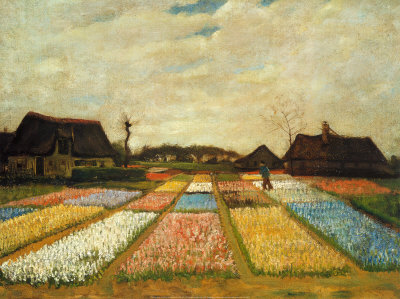## Math Puzzle: Where is The Missing Square?

If you have already found answer to the missing area puzzle, you might want to try the mysterious missing square below. The largest triangle in the first figure is made up of two smaller triangles and two L-shaped polygons.The largest triangle below (well, technically it’s not really a triangle) is also made up of the same polygons mentioned above. » Read more

## Math Puzzle: Where is the Missing Area?

The square below is made up of two triangles and two trapezoids. The dimensions of the square is$13$ by$13$, which means that the area is$169$ square units.The same polygons above are used to create the rectangle below. The dimensions of the rectangle is$8$ by$21$, which means that the area is$168$. » Read more

## The Mathematics of Perspective Drawing

Painters use different techniques to create realistic paintings. Vincent Van Gogh’s Flower Beds in Holland is one of the examples of such.  Given the right distance, you would mistake it for a faded photograph.

But how do painters put so much life in their works? How do they put real space (3 dimensions) on a flat surface (2 dimension)?One of the techniques used by painters is called perspective.   Perspective is the art of drawing objects in such a way so as to give them depth and show their distance from the observer. This gives a drawing the illusion  of three-dimensional space. » Read more

1 2 3 4 5 6 9